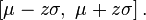# Basic Statistics

Basic Variables to look at when attempting to discover a new set:

N
Mean

SE Mean

StDev

Variance

CoefVar

Minimum

Q1

Median

Q3

Maximum

Range

Skewness

95% range limits: Mean ± 1.95*StdDev.

Confidence interval: The range in which we are x% sure that the population mean (also the regression line) will fall in.Prediction interval: the range in which we are x% sure that all points will fall in. It is and not influenced by the sample size n.Statistical Model Analysis: Regression as an example:Satistics Plots